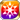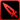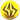# Inherited Iron Will

A precious book, a precious time. Moments spent bonding with my dad like this were very special to me. My heart longed not for the hero of the story, but for the smiling man seated next to me. I was innocent then, answering my father's teachings with childish naivete. "What do I want to protect? Hmm... If I have something like that, d'you think I'll be able to grow up to be like you, Dad?" That question was my foundation; the source of the iron will that I inherited from my dad. Together with that faithful old set of armor—dented and scored though it may be—my resolve drove me to keep moving forward, aiming always to follow in his footsteps. (Found scrawled among the pages of Gerald's favorite book)

### Inherited Iron Will

Raises PATK by 40%/Area ATK Power by 20%/PDEF by 30%/AGI by 10%/Strong vs Wrath by 40%/Strong vs Gluttony by 20% for the Memento group "Scarlet Flame Guard"

Target Buff (SelfSide) (CardLsSkill)
Stats
TypeMinMax
PATK+40%+40%
Area ATK+20+20
PDEF+30%+30%
AGI+10%+10%
Strong vs <憤怒>+40+40
Strong vs <暴食>+20+20

## Stats

#### Simple stats

TypeLvl 1Lvl 30Lvl 40
HP+30+67+80

LevelHP
1+30
2+31
3+32
4+33
5+35
6+36
7+37
8+38
9+40
10+41
LevelHP
11+42
12+44
13+45
14+46
15+47
16+49
17+50
18+51
19+53
20+54
LevelHP
21+55
22+56
23+58
24+59
25+60
26+62
27+63
28+64
29+65
30+67
LevelHP
31+68
32+69
33+71
34+72
35+73
36+74
37+76
38+77
39+78
40+80

## Group skills

### Envylia's Defense

#### Simple stats

TypeLvl 1Lvl 30Lvl 40
PDEF+15+41+50

LevelPDEF
1+15
2+15
3+16
4+17
5+18
6+19
7+20
8+21
9+22
10+23
LevelPDEF
11+23
12+24
13+25
14+26
15+27
16+28
17+29
18+30
19+31
20+32
LevelPDEF
21+32
22+33
23+34
24+35
25+36
26+37
27+38
28+39
29+40
30+41
LevelPDEF
31+41
32+42
33+43
34+44
35+45
36+46
37+47
38+48
39+49
40+50

#### Limit Break

TypeLB1LB2LB3LB4LB5
PDEF+6+12+18+24+30

### Envylia's Defense

#### Simple stats

TypeLvl 1Lvl 30Lvl 40
PDEF+15+41+50

LevelPDEF
1+15
2+15
3+16
4+17
5+18
6+19
7+20
8+21
9+22
10+23
LevelPDEF
11+23
12+24
13+25
14+26
15+27
16+28
17+29
18+30
19+31
20+32
LevelPDEF
21+32
22+33
23+34
24+35
25+36
26+37
27+38
28+39
29+40
30+41
LevelPDEF
31+41
32+42
33+43
34+44
35+45
36+46
37+47
38+48
39+49
40+50

#### Limit Break

TypeLB1LB2LB3LB4LB5
PDEF+6+12+18+24+30

### Burning Resolve

#### Simple stats

TypeLvl 1Lvl 30Lvl 40
Initial Jewels+3+8+10
Paralyze Res+10+24+30

#### Detailed stats

LevelInitial JewelsParalyze Res
1+3+10
2+3+10
3+3+11
4+3+11
5+3+12
6+3+12
7+4+13
8+4+13
9+4+14
10+4+14
LevelInitial JewelsParalyze Res
11+4+15
12+4+15
13+5+16
14+5+16
15+5+17
16+5+17
17+5+18
18+6+18
19+6+19
20+6+19
LevelInitial JewelsParalyze Res
21+6+20
22+6+20
23+6+21
24+7+21
25+7+22
26+7+22
27+7+23
28+7+23
29+8+24
30+8+24
LevelInitial JewelsParalyze Res
31+8+25
32+8+25
33+8+26
34+8+26
35+9+27
36+9+27
37+9+28
38+9+28
39+9+29
40+10+30

#### Limit Break

TypeLB1LB2LB3LB4LB5
Initial Jewels+1+2+3+4+6
Paralyze Res+6+9+12+15+18

### Burning Resolve

#### Simple stats

TypeLvl 1Lvl 30Lvl 40
Initial Jewels+3+8+10
Paralyze Res+10+24+30

#### Detailed stats

LevelInitial JewelsParalyze Res
1+3+10
2+3+10
3+3+11
4+3+11
5+3+12
6+3+12
7+4+13
8+4+13
9+4+14
10+4+14
LevelInitial JewelsParalyze Res
11+4+15
12+4+15
13+5+16
14+5+16
15+5+17
16+5+17
17+5+18
18+6+18
19+6+19
20+6+19
LevelInitial JewelsParalyze Res
21+6+20
22+6+20
23+6+21
24+7+21
25+7+22
26+7+22
27+7+23
28+7+23
29+8+24
30+8+24
LevelInitial JewelsParalyze Res
31+8+25
32+8+25
33+8+26
34+8+26
35+9+27
36+9+27
37+9+28
38+9+28
39+9+29
40+10+30

#### Limit Break

TypeLB1LB2LB3LB4LB5
Initial Jewels+1+2+3+4+6
Paralyze Res+6+9+12+15+18

### Pledge to Father

#### Simple stats

TypeLvl 1Lvl 30Lvl 40
HP+130+330+400
PATK+20+49+60
Thunder+3+8+10

#### Detailed stats

LevelHPPATKThunder
1+130+20+3
2+136+21+3
3+143+22+3
4+150+23+3
5+157+24+3
6+164+25+3
7+171+26+4
8+178+27+4
9+185+28+4
10+192+29+4
LevelHPPATKThunder
11+199+30+4
12+206+31+4
13+213+32+5
14+220+33+5
15+226+34+5
16+233+35+5
17+240+36+5
18+247+37+6
19+254+38+6
20+261+39+6
LevelHPPATKThunder
21+268+40+6
22+275+41+6
23+282+42+6
24+289+43+7
25+296+44+7
26+303+45+7
27+310+46+7
28+316+47+7
29+323+48+8
30+330+49+8
LevelHPPATKThunder
31+337+50+8
32+344+51+8
33+351+52+8
34+358+53+8
35+365+54+9
36+372+55+9
37+379+56+9
38+386+57+9
39+393+58+9
40+400+60+10

#### Limit Break

TypeLB1LB2LB3LB4LB5
HP+48+96+144+192+240
PATK+7+14+21+28+36
Thunder+1+2+3+4+6

TypeLB5
AGI+10

## Vision abilities

### Limit Reached Bonus

#### Ability base

• Slot:Support

Revealed after Limit Reached. Raises AGI after appearing on map.

#### Ability max

• Slot:Action

Raises AGI after appearing on map.

#### Ability base

• Slot:Action
• DMG Type:Physical
• ATK Type:Pierce
• Element:Thunder

Thunder Pierce ATK on enemy units within area & chance of critical & lowers Thunder Res for three turns [Range: 4, Area: Line (4), Height Range: 2]

Skill Effect
TypeMinMax
Attack100%129%
• Charges: 2
• Jewel Cost: 30
• Height: 2
• Select Range: Laser
• Range: 0-4
• Select Scope: Laser
• Scope: 1

#### Ability max

• Slot:Action
• DMG Type:Physical
• ATK Type:Pierce
• Element:Thunder

Thunder Pierce ATK on enemy units within area & chance of critical & lowers Thunder Res for three turns (Stackable up to 2x) [Range: 4, Area: Line (4), Height Range: 2]

Skill Effect
TypeMinMax
Attack167%167%
• Charges: 2
• Jewel Cost: 30
• Height: 2
• Select Range: Laser
• Range: 0-4
• Select Scope: Laser
• Scope: 1

#### Unit and job restriction

[[Category:Strong vs <憤怒>]][[Category:Strong vs <暴食>]]